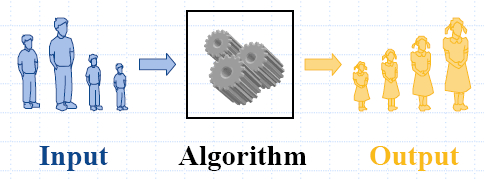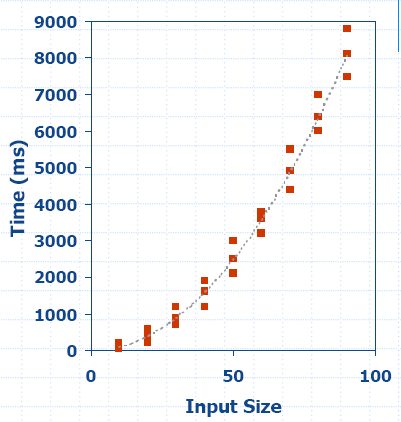## Chapter 3: Analysis Tools

Analysis.pdf

####• An algorithm is a step-by-step procedure for solving a problem in a finite amount of time.
• Most algorithms transform input objects into output objects.

#### Experimental Studies

• Write a program implementing the algorithm.
• Run the program with inputs of varying size and composition.
• Use a function, like the built-in clock() function, to get an accurate measure of the actual running time.
• Plot the results.### Limitations of Experiments

• It is necessary to implement the algorithm, which may be difficult.
• Results may not be indicative of the running time on other inputs not included in the experiment.
• In order to compare two algorithms, the same hardware and software environments must be used.

### Running Time

• The running time of an algorithm typically grows with the input size.
• Average case time is often difficult to determine.
• We focus on the worst case running time.
• Easier to analyze.
• Crucial to applications such as games, finance and robotics.

### Theoretical Analysis

• Uses a high-level description of the algorithm instead of an implementation.
• Characterizes running time as a function T(n) of the input size, n.
• Takes into account all possible inputs.
• Allows us to evaluate the speed of an algorithm independent of the hardware/software environment.

### Pseudocode

• Pseudocode is a program code, unrelated to the hardware of a particular computer.
• It requires conversion to the code used by the computer before the program can be used.
• No strict syntax rules, designed for humans, not for computers.
• High-level description of an algorithm.
• More structured than English prose.
• Less detailed than a program.
• Preferred notation for describing algorithms.
• Hides program design issues.
Example: Find max element of an array.

 Algorithm arrayMax(A, n)   Input: array A of n integers   Output: maximum element of A   currentMax <- A   for i <- 1 to n−1 do     if A[i] > currentMax then currentMax <- A[i]   return currentMax

### Pseudocode Details

• Control flow
if…then…[else…]
while…do…
repeat…until…
for…do…

Indentation replaces braces

• Method declaration
Algorithm method(arg[, arg…])
Input…
Output…
• Method/Function call
var.method (arg[, arg…])
• Return value
return expression
• Expressions
<- Assignment (like = in C++)
= Equality testing (like == in C++)
n2 Superscripts and other mathematical formatting allowed.

### The Random Access Machine - (RAM) Model

• A CPU.
• An potentially unbounded bank of memory cells, each of which can hold an arbitrary number or character.
• Memory cells are numbered and accessing any cell in memory takes unit time.

### Primitive Operations

• Basic computations performed by an algorithm.
• Identifiable in pseudocode.
• Largely independent from the programming language.
• Exact definition not important (we will see why later).
• Assumed to take a constant amount of time in the RAM model.
Examples:
• Evaluating an expression.
• Assigning a value to a variable.
• Indexing into an array.
• Calling a method.
• Returning from a method.

### Counting Primitive Operations

• By inspecting the pseudocode, we can determine the maximum number of primitive operations executed by an algorithm, as a function of the input size n.
 Algorithm arrayMax(A, n) Number of operations currentMax <- A 2 for i <- 1 to n−1 do 2 + n if A[i] > currentMax then 2(n − 1) currentMax <- A[i] 2(n − 1) { increment counter i} 2(n −1) return currentMax 1 Total 7n − 1

### Estimating Running Time

• Algorithm arrayMax executes 7n−1 primitive operations in the worst case. Define:
a = time taken by the fastest primitive operation.
b = time taken by the slowest primitive operation.
• Let T(n) be worst-case time of arrayMax. Then
a (7n − 1) ≤ T(n) ≤ b(7n − 1).
• Hence, the running time T(n) is bounded by two linear functions.

### Growth Rate of Running Time

Changing the hardware/ software environment
• effects T(n) by a constant factor, but
• does not alter the growth rate of T(n).
The linear growth rate of the running time T(n) is an intrinsic property of the algorithm arrayMax.

### Growth Rates

 Function / n 1 2 10 100 1000 5 5 5 5 5 5 log n 0 1 3.32 6.64 9.96 n 1 2 10 100 1000 n log n 0 2 33.2 664 9996 n2 1 4 100 104 106 n3 1 8 1000 106 109 2n 2 4 1024 1030 10300 n! 1 2 3.6288e+06 10157 102567 nn 1 4 1010 10200 103000

### Constant Factors

The growth rate is not affected by
• constant factors or
• lower-order terms.
Examples:
• 102n+105 is a linear function.
• 105n2+108n is a quadratic function.

### Asymptotic Notation

Big-Oh

Given functions f(n) and g(n), we say that f(n) is O(g(n)) if there are positive constants c and N > 0 such that f(n) < c g(n) for n > N.

Example 1
: 2n +10 is O(n).
2n +10 ≤ cn; (c − 2) n ≥10; n ≥ 10/(c − 2).
Pick c = 3 and N = 10.

Example 2
: the function n2 is not O(n).
n2c n; nc
The above inequality cannot be satisfied since c must be a constant.

Example 3
: 7n - 2 is O(n).
need c > 0 and N ≥ 1 such that 7n - 2 ≤ c n for nN,
this is true for c = 7 and N = 1.

Example 4
: 3n3+ 20n2+ 5 is O(n3)
Need c > 0 and N ≥ 1 such that 3n3+ 20n2+ 5 ≤ cn3 for nN,
this is true for c = 4 and N = 21.

Example 5
: 3 log n + log log n is O(log n)
Need c > 0 and N ≥ 1 such that 3 log n + log log nc log n for nN,
this is true for c = 4 and N = 2.

### Big-Oh Rules

• If is f(n) a polynomial of degree d, then f(n) is O(nd), i.e.
1. drop lower-order terms;
2. drop constant factors.
• Use the smallest possible class of functions.
Say “2n is O(n)” instead of  “2n is O(n2)”.
• Use the simplest expression of the class.
Say “3n + 5 is O(n)” instead of  3n + 5 is O(3n).

### Asymptotic Algorithm Analysis

• The asymptotic analysis of an algorithm determines the running time in big-Oh notation.
• To perform the asymptotic analysis:
1. We find the worst-case number of primitive operations executed as a function of the input size.
2. We express this function with big-Oh notation.
Example:
We determine that algorithm
arrayMax executes at most 7n−1 primitive operations.
We say that algorithm
arrayMax “runs in O(n) time”.
• Since constant factors and lower-order terms are eventually dropped anyhow, we can disregard them when counting primitive operations.

### Computing Prefix Averages

• We further illustrate asymptotic analysis with two algorithms for prefix averages.
• The i-th prefix average of an array X is average of the first (i+1) elements of X:
A[i]= (X +X +… +X[i])/(i+1)
• Computing the array A of prefix averages of another array X has applications to financial analysis.

The following algorithm computes prefix averages in quadratic time by applying the definition:
 Algorithm prefixAverages1(X, n) Input array X of n integers Output array A of prefix averages of X A <- new array of n integers for i <- 0 to n−1 do    s <- X    for j <- 1 to i do       s <- s+X[j]    A[i] <- s/(i+1) return A #operations n n n 1 + 2 + …+(n−1) 1 + 2 + …+(n−1) n 1

### Arithmetic Progression

• The running time of prefixAverages1 is O(1 + 2 + …+ n).
• The sum of the first n integers is n(n + 1) / 2.
• Thus, algorithm prefixAverages1 runs in O(n2) time.

### Prefix Averages (Linear)

The following algorithm computes prefix averages in linear time by keeping a running sum.

 Algorithm prefixAverages2(X, n) Input array X of n integers Output array A of prefix averages of X A <- new array of n integers s <- 0 for i <- 0 to n−1 do    s <- s + X[i]    A[i] <- s/(i + 1) return A #operations n 1 n n n 1

Algorithm prefixAverages2 runs in O(n) time.

### Relatives of Big-Oh

Big-Omega
f(n) is
Ω(g(n)) if there is a constant c > 0 and an integer constant N > 0 such that f(n) > c g(n) for n > N.

Big-Theta
f(n) is
Θ(g(n)) if there are constants c' > 0 and c" > 0 and an integer constant N > 0 such that c'g(n) <  f(n) < c"g(n) for n > N.

little-oh
f(n) is o(g(n)) if, for any constant c > 0, there is an integer constant N > 0 such that f(n) < c g(n) for n > N.

little-omega
f(n) is
ω(g(n)) if, for any constant c > 0, there is an integer constant N > 0 such that f(n) < c g(n) for n > N.

### Intuition for Asymptotic Notation

Big-Oh
f(n) is O(g(n)) if f(n) is asymptotically less than or equal to g(n).

big-Omega
f(n) is Ω(g(n)) if f(n) is asymptotically greater than or equal to g(n).

big-Theta
f(n) is Θ(g(n)) if f(n) is asymptotically equal to g(n).

little-oh
f(n) is o(g(n)) if f(n) is asymptotically strictly less than g(n).

little-omega
f(n)) is ω(g(n)) if f(n) is asymptotically strictly greater than g(n).

Example Uses of the Relatives of Big-Oh

Example 1: 5n2 is Ω(n
2).
f(n) is Ω(g(n)) if there is a constant c > 0 and an integer constant N ≥ 1 such that f(n) ≥ c g(n) for nN,
let c = 5 and N = 1.

Example 2:
5n2 is Ω(n).
f(n) is Ω(g(n)) if there is a constant c > 0 and an integer constant N ≥ 1 such that f(n) ≥c g(n) for nN,
let c = 1 and N = 1,
5n n.

Example 3: 5n2 is ω(n).
f(n) is ω(g(n)) if, for any constant c > 0, there is an integer constant N ≥ 0 such that f(n) ≥c g(n) for nN,
need 5N2cN - given c, then N that satisfies Nc/5 ≥ 0.